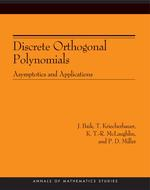# Discrete Orthogonal Polynomials. (AM-164): Asymptotics and Applications (AM-164)

J. Baik
T. Kriecherbauer
K. T.-R. McLaughlin
P. D. Miller
Pages: 184
https://www.jstor.org/stable/j.ctt2854np

Export Selected Citations Export to NoodleTools Export to RefWorks Export to EasyBib Export a RIS file (For EndNote, ProCite, Reference Manager, Zotero, Mendeley...) Export a Text file (For BibTex)
1. Front Matter
(pp. i-iv)
(pp. v-vi)
3. Preface
(pp. vii-viii)
4. Chapter One Introduction
(pp. 1-24)

The main aim of this monograph is to deduce asymptotic properties of polynomials that are orthogonal with respect to pure point measures supported on finite sets and use them to establish various statistical properties of discrete orthogonal polynomial ensembles, a special case of which yields new results for a random rhombus tiling of a large hexagon. Throughout this monograph, the polynomials that are orthogonal with respect to pure point measures will be referred to simply asdiscrete orthogonal polynomials. We begin by introducing several applications in which asymptotics of discrete orthogonal polynomials play an important role.

In order to illustrate...

5. Chapter Two Asymptotics of General Discrete Orthogonal Polynomials in the Complex Plane
(pp. 25-48)

In this chapter we state our results for the asymptotics of the discrete orthogonal polynomials subject to the conditions described in the introduction, in the limit$N\hspace{3pt}\rightarrow\hspace{3pt}\infty$. The asymptotic formulae we will present characterize the polynomials in terms of an equilibrium measure (described below in §2.1) and also the function theory of a hyperelliptic Riemann surface associated with the equilibrium measure (described below in §2.2). The results themselves will follow in §2.3.

It has been recognized for some time (see [Rak96, DraS97], as well as the review article [KuiR98]), that the asymptotic behavior of discrete orthogonal polynomials in the...

6. Chapter Three Applications
(pp. 49-66)

Recall from §1.1.1 that the discrete orthogonal polynomial ensemble is the statistical ensemble associated with the density (1.1):

$p^{\left(N,k\right)}\left(x_{1},...,x_{k}\right):=\mathbb{P}\left(\text{there are particles at each of the nodes}\hspace{5pt}x_{1},...,x_{k}\right)\\\hspace{170pt}=\hspace{3pt}\frac1{Z_{N,k}}\hspace{3pt}\prod_{1\le{i}\lt{j}\le{k}}\left(x_{i}-x_{j}\right)^{2}.\ \prod_{j=1}^{k}w\left(x_{j}\right),\quad{(3.1)}$

where$x_{1},...,x_{k}\hspace{3pt}\in\hspace{3pt}X_{N},$and$X_{N}$is a discrete set of cardinality$N$as described in §1.2.

Some common properties of discrete orthogonal polynomial ensembles can be read off immediately from formula (3.1). For example, the presence of the Vandermonde factor means that the probability of finding two particles at the same site in$X_{N}$is zero. Thus a discrete orthogonal polynomial ensemble always describes an exclusion process. This phenomenon is the discrete analogue of the familiarlevel repulsionphenomenon in random matrix...

7. Chapter Four An Equivalent Riemann-Hilbert Problem
(pp. 67-86)

In this chapter we introduce a sequence of exact transformations relating the matrix$\text{P}\left(z;\hspace{3pt}N,\hspace{3pt}k\right)$to a matrix$\text{X}\left(z\right)$satisfying an equivalent Riemann-Hilbert problem. The Riemann-Hilbert problem characterizing the matrix$\text{X}\left(z\right)$will be amenable to asymptotic analysis in the joint limit of large degree$k$and large parameter$N$. This asymptotic analysis will be carried out in Chapter 5.

It turns out that Interpolation Problem 1.2 will be amenable to analysis without any modification of the triangularity of some of the residue matrices only if the equilibrium measure never realizes its upper constraint. This is because the variational inequality (2.16)...

8. Chapter Five Asymptotic Analysis
(pp. 87-104)

In this chapter we provide all the tools for a complete asymptotic analysis of discrete orthogonal polynomials with a large class of (generally nonclassical) weights, in the joint limit of large degree and a large number of nodes. These results will then be used in Chapter 6 to establish precise convergence theorems about the discrete orthogonal polynomials, and in Chapter 7 to prove a number of universality results concerning statistics of related discrete orthogonal polynomial ensembles.

Our immediate goal is to use the deformations we have carried out to construct a model for the matrix$\text{X}\left(z\right)$that we expect to...

9. Chapter Six Discrete Orthogonal Polynomials: Proofs of Theorems Stated in §2.3
(pp. 105-114)

In this chapter, we start with the exact formula for$\text{X}\left(z\right)$valid in the entire complex$z$-plane:

$\text{X}\left(z\right)\hspace{3pt}=\hspace{3pt}\text{E}\left(z\right)\text{\hat{X}}\left(z\right). (6.1)$

Thus$\text{X}\left(z\right)$is written in terms of the explicit global parametrix and the matrix$\text{E}\left(z\right)$, which while not explicit is characterized by Proposition 5.15. We then work backward to the matrix$\text{P}\left(z;\hspace{3pt}N,\hspace{3pt}k\right)$and thereby obtain exact formulae for the monic polynomials$\pi_{N,\hspace{3pt}k}\left(z\right)$valid in the whole complex plane, as well as the normalization constants$\gamma_{N,\hspace{3pt}k}$and recurrence coefficients$a_{N,\hspace{3pt}k}$and$b_{N,\hspace{3pt}k}$, in terms of the matrix elements of$\hat{\text{X}}\left(z\right)$and$\text{E}\left(z\right)$, and their asymptotics for large$z$....

10. Chapter Seven Universality: Proofs of Theorems Stated in §3.3
(pp. 115-134)

Since the holes are also governed by a discrete orthogonal polynomial ensemble, the correlation functions for holes are again represented as determinants involving the reproducing kernel, this time corresponding to the dual weights. It turns out that there is a simple relation between the correlation functions for particles and those for holes.

Let$\overline{R}_{m}^{\left(N,\overline{k}\right)}$be the$m$-point correlation function of the dual orthogonal polynomial ensemble for the holes. Hence$\overline{R}_{m}^{\left(N,\overline{k}\right)}$is defined as in (3.2), with the replacement of$p^{\left(N,k\right)}$by$\overline{p}^{\left(N,\overline{k}\right)}$. Let$\overline{K}_{N,\overline{k}}$denote the reproducing kernel of the dual ensemble. Then (3.3) implies that

$\overline{R}_{m}^{\left(N,\overline{k}\right)}\left(x_{1},...,x_{m}\right)\hspace{3pt}=\hspace{3pt}\det \left(\overline{K}_{N,\overline{k}}\left(x_{i},x_{j}\right)\right)_{1 \le i,j \le m},$

for...

11. Appendix A. The Explicit Solution of Riemann-Hilbert Problem 5.1
(pp. 135-144)
12. Appendix B. Construction of the Hahn Equilibrium Measure: the Proof of Theorem 2.17
(pp. 145-152)
13. Appendix C. List of Important Symbols
(pp. 153-162)
14. Bibliography
(pp. 163-166)
15. Index
(pp. 167-170)